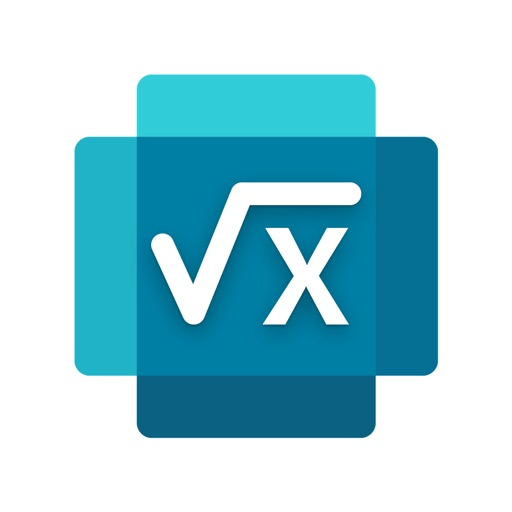## Making Math Easy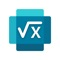# Microsoft Math Solver

by Microsoft Corporation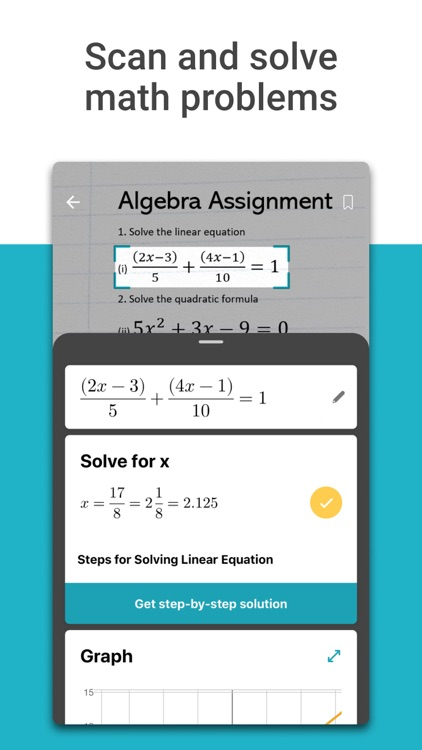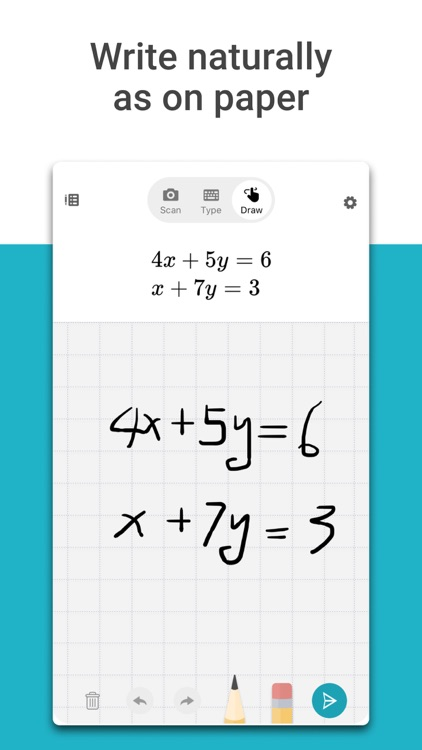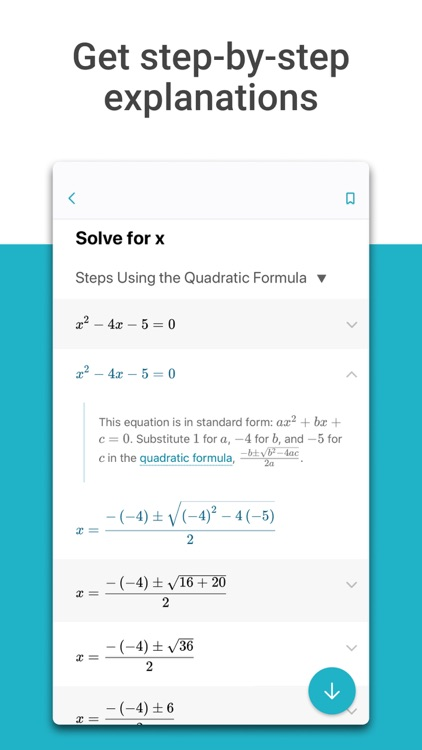Microsoft Math solver app provides help with elementary, middle and high school math problems by leveraging an advanced AI powered math solver. Simply write a math problem on screen or use the camera to snap a photo of a math problem. Microsoft Math instantly recognizes the problem and helps you to solve it with step-by-step explanation, interactive graphs, similar problems from the web and online video lectures. Practice new problems with automatically generated worksheets. Quickly look up related math concepts. Get help with your homework problems and gain confidence in mastering the techniques with Microsoft Math. It's absolutely FREE!!!### App Details

Version
1.0.2
Rating
(498)
Size
32Mb
Genre
Education Utilities
Last updated
November 27, 2019
Release date
November 21, 2019

### App Screenshots### App Store Description

Microsoft Math solver app provides help with elementary, middle and high school math problems by leveraging an advanced AI powered math solver. Simply write a math problem on screen or use the camera to snap a photo of a math problem. Microsoft Math instantly recognizes the problem and helps you to solve it with step-by-step explanation, interactive graphs, similar problems from the web and online video lectures. Practice new problems with automatically generated worksheets. Quickly look up related math concepts. Get help with your homework problems and gain confidence in mastering the techniques with Microsoft Math. It's absolutely FREE!!!

HIGHLIGHTS
● Draw a math equation on screen as you naturally do on paper [supports Apple Pencil]
● Scan printed or handwritten photo of math equation
● Type and edit using advanced scientific math calculator
● Get interactive Step-by-Step solutions & Graphs
● Import images with math equations from camera roll
● Scan and Solve Math Worksheets with multiple problems
● Search the web for similar problems and video lectures
● Generate printable Practice Worksheets
● Try math word problems
● Scan and plot x-y data tables for linear/non-linear functions
● Get help in multiple languages

SUPPORTED PROBLEMS
● Elementary: arithmetic, real, complex numbers, LCM, GCD, factors, roman numerals
● Pre-Algebra: radicals and exponents, fractions, matrices, determinants
● Algebra: quadratic equations, system of equations, inequalities, rational expressions, linear, quadratic and exponential graphs
● Word problems on math concepts, number theory, probability, volume, surface area
● Basic Calculus: Summations, Limits, derivatives, integrals
● Statistics: Mean, Median, Mode, Standard Deviation, permutations, combinations